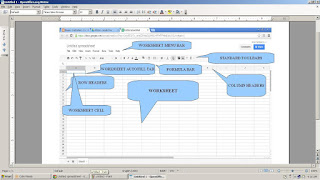Parts Of Google Drive Spreadsheets

The Basic Parts: See the image shown above

1. Worksheet Menu bar- located above the standard toolbar and below the worksheet title untitled spreadsheets. Use this for any command application in your worksheet or spreadsheet data.
2. Standard Toolbar- located below the Menu bar, this is use for standard application or commonly use command application using the buttons. One of the most common button use is the borders where you can create a worksheet table of your spreadsheet page.
3. Formula bar- this is use to compose logical formula base of your spreadsheet data. This is use also to  edit a certain data inside your worksheet. Just click the data you want to edit and click the formula bar. You can now erase and type the data you want to replace. It is situated below the standard toolbar.
4. Worksheet Cell- use as the reference part of the data, if want to insert data inside your worksheets just move the cell using the arrow keys or click your mouse where you want to insert your data within your worksheet table.
5. Worksheet Autofill Tab- this part is built-in of the worksheet cell, this is to auto execute the calculation within the worksheet data table. So, if you add a data base of the column and row of the worksheet table, the preceding data is no need to add again. All you have to do is to point your mouse on the worksheet autofill tab and drag down your mouse using the left button.
6. Column Headers- column header is use to indicate the data within the worksheet or worksheet table. This is the vertical gridlines found inside the worksheet The standard worksheet have a 256 columns. You need to combine the column and rows intersection so that you can calculate the data inside of it., ex: A1 + B1.
7. Row Headers- use to indicate the data inside the row section of the worksheet. This is the horizontal gridlines found inside the worksheet or worksheet table. The standard numbers of the row are more than 64 thousand. You need to combine the column and rows intersection so that you can calculate the data inside of it., ex: A1 + B1.
8. Worksheet Area or Worksheet- this is the biggest part of the Google Drive spreadsheet. This is where you can insert and type your data and perform calculation. The worksheet is the simulation of the paper spreadsheet where the column and rows are combine together using the gridlines of vertical and horizontal lines base of intersection to perform certain function or logical calculation.
That's all the part of this online spreadsheet of the Google Drive. Just study first the parts and function before you proceed any application. Or just follow this blog for the next update of application. " End"